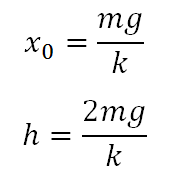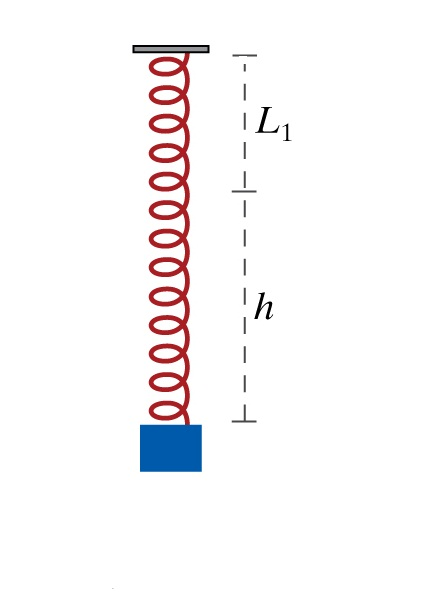# Maximum displacement of a block hanging from a spring Calculator

This CalcTown calculator calculates the mean position and maximum displacement of a block hanging from a spring from its unstretched length.

N/m
Kg

#### Result

m
mClick here to view image

where

x0=Mean Elongation in the spring

h=Maximum Displacement of the bob from its mean position